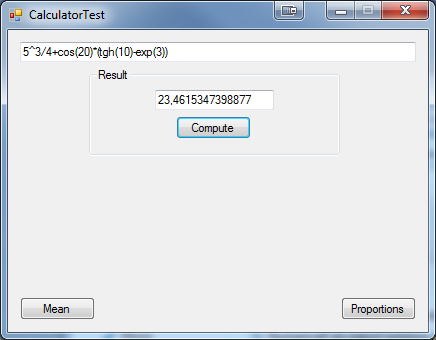### Features

You can try Mean and Proportion functionality below.

Functions which library can handle:
• Sine = sin
• Hyperbolic sine = sinh
• Cosine = cos
• Hyperbolic cosine = cosh
• Tangent = tg, tan
• Hyperbolic tangent = tgh, tanh
• Cotangent = ctg, ctn, cot
• Hyperbolic cotangent = ctgh, ctnh, coth
• Secant = sec
• Cosecant = csc
• Arcsine = asin
• Arccosine = acos
• Arctangent = atg
• Arccotangent = actg
• Exponential function with e base = exp
• Square root = sqrt
• Base 2 logarithm = lg
• Base e logarithm = ln
• Base 10 logarithm = log
Factorial of natural numbers less then 20 is computed with standard formula:
5! = 1*2*3*4*5
Factorial of real numbers and natural numbers greater than 19 is gamma function.

You can use sinx instead of sin(x) but it's not recommended - in some cases it can affect the result.
For example sinx+2 is sin(x)+2 not sin(x+2).

In formulas you can use PI and E. PI will be calculated as 3.14159265358979,
and E as 10 raised to the power of that what is after E.
For example: E-05 = 10^(-5)

Spaces in formulas are ignored.

Application can't compute imaginary numbers. If during computations
Numerical Calculator encounter imaginary numbers it will return "not a number" result.

You can input nested expressions like sin(cos(30)+tg(sqrt(0,4)))

### Algorithm

Library uses reverse polish notation to understand formulas. It splits input into separate functions then taking into account order of operations reorder them and computes.

### Usage

`string formula = "5^3/4+cos(20)*(tgh(10)-exp(3))";Calculator calc = new Calculator(formula);double result = calc.Compute();`

### Example application